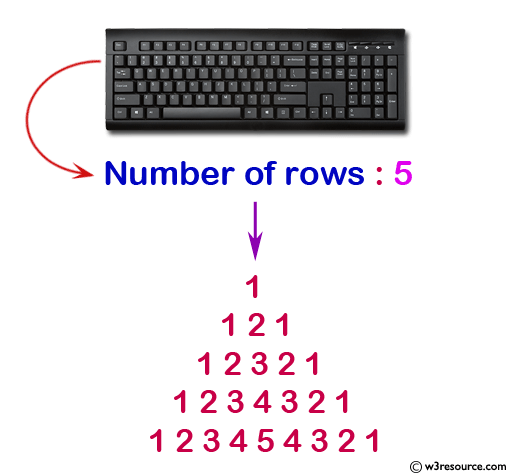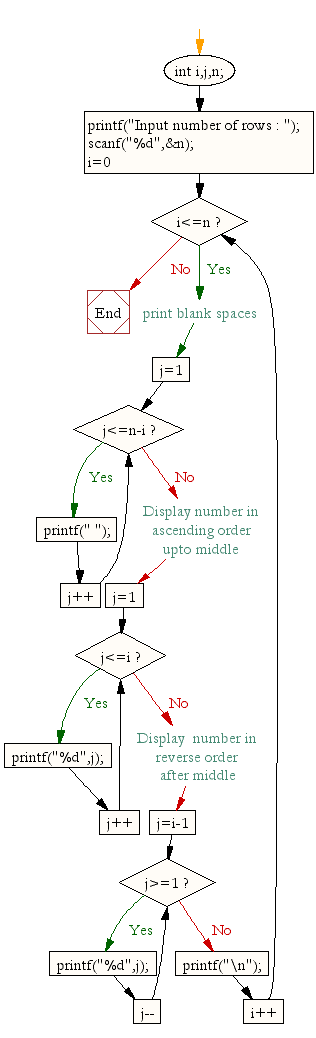﻿ C : Pattern with 1 as the first and last number of each row# C Exercises: Display the pattern in which the first and a last number of each row will be 1

## C For Loop: Exercise-36 with Solution

Write a program in C to display the such a pattern for n number of rows using a number which will start with the number 1 and the first and a last number of each row will be 1. The pattern is as follows:

```   1
121
12321
```

Pictorial Presentation:Sample Solution:

C Code:

``````#include <stdio.h>

void main()
{
int i,j,n;
printf("Input number of rows : ");
scanf("%d",&n);
for(i=0;i<=n;i++)
{
/* print blank spaces */
for(j=1;j<=n-i;j++)
printf(" ");
/* Display number in ascending order upto middle*/
for(j=1;j<=i;j++)
printf("%d",j);

/* Display  number in reverse order after middle */
for(j=i-1;j>=1;j--)
printf("%d",j);

printf("\n");
}
}
```
```

Sample Output:

```Input number of rows : 5

1
121
12321
1234321
123454321
```

Flowchart:C Programming Code Editor:

Improve this sample solution and post your code through Disqus.

What is the difficulty level of this exercise?

Test your Programming skills with w3resource's quiz.

﻿

## C Programming: Tips of the Day

How to initialize array to 0 in C?

Global variables and static variables are automatically initialized to zero. If you have simply-

`char ZEROARRAY;`

at global scope it will be all zeros at runtime. But actually there is a shorthand syntax if you had a local array. If an array is partially initialized, elements that are not initialized receive the value 0 of the appropriate type. You could write:

`char ZEROARRAY = {0};`

The compiler would fill the unwritten entries with zeros. Alternatively you could use memset to initialize the array at program startup:

Ref : https://bit.ly/3cvqplP